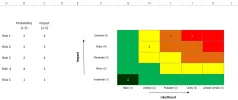# ExcelPlotting heat-map

#### Tahir M

Hello,

I am trying to plot a heat map using two columns (Likelihood and Impact) both have range from 1 to 5. Is there any formula to show sum of combinations as show in attachment.

Regards,
Tahir

#### Attachments

•to b attached.PNG
22.1 KB · Views: 95

#### Becky

##### Webmistress
I'm not sure I understand what you're asking... Do you want a formula that tells you the risk level if likelihood and impact are specified?

#### Tahir M

Thanks Becky for quick response.

No, I want a formula which adds the number of points using column B and C (of attached sheet's, snapshot). and show the sum on specific coordinate of heat-map (Matrix with different colors as shown).
I have manually done by taking example of 5 risks.

There are two risks with probability 2 and Impact 4 (Risk 1 and Risk 3) so "2" is being displayed on coordinates 2,4 (Likelihood, Impact), Similarly there is one risk (Risk 2) with probability 3 and Impact 5, so 1 is appearing on coordinate 3,5 and so on...

The numbers shown on matrix are entered manually. We have long lists and therefore looking for a formula to plot the data on matrix automatically when values in column B and C are entered.

Please let me know if further clarification is required.

#### Becky

##### Webmistress
I don't know of a way to do this I'm afraid. Is it necessary to plot the point or would it be sufficient to just give the risk profile (given the probability and impact)?